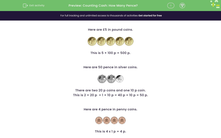# Counting Cash: How Many Pence?

In this worksheet, students recognise the given coins and work out the total value in pence.Key stage:  KS 2

Curriculum topic:   Measurement

Popular topics:   Money worksheets, Counting worksheets

Difficulty level:#### Worksheet Overview

Here are £5 in pound coins.This is 5 × 100 p = 500 p.

Here are 50 pence in silver coins.There are two 20 p coins and one 10 p coin.

This is 2 × 20 p  + 1 × 10 p = 40 p + 10 p = 50 p.

Here are 4 pence in penny coins.This is 4 x 1 p = 4 p.

Altogether, we have 500 p + 50 p + 4 p = 554 p.

This is also £5.54.

We say that:

£5.54 is the same as 554 p.

### What is EdPlace?

We're your National Curriculum aligned online education content provider helping each child succeed in English, maths and science from year 1 to GCSE. With an EdPlace account you’ll be able to track and measure progress, helping each child achieve their best. We build confidence and attainment by personalising each child’s learning at a level that suits them.

Get started